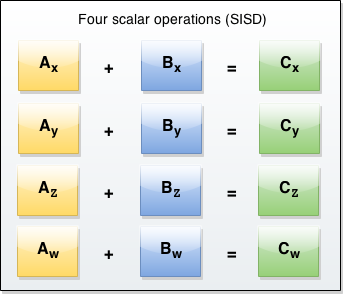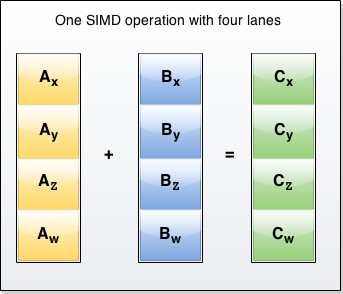# SIMD

SIMD (pronounced "sim-dee") is short for Single Instruction/Multiple Data which is one classification of computer architectures. SIMD operations perform the same computation on multiple data points resulting in data level parallelism and thus performance gains, for example for 3D graphics and video processing, physics simulations or cryptography, and other domains.

This page and sub pages are the SIMD API reference documentation. See also SIMD types for an article that describes SIMD in JavaScript more generally.

## Description

The JavaScript SIMD API consists of several new types and operations. Browsers provide highly optimized implementations of this API depending on the underlying hardware of the user. Currently, SIMD is especially modeled for ARMv7 platforms with NEON and x86 platforms with SSE.

The SIMD API types are installed on a `SIMD` module. Unlike the other global objects, `SIMD` is not a constructor. You can not use it with a `new` operator or invoke the `SIMD` object as a function. All properties and methods of `SIMD` are static (as is the case with the `Math` object).

### Overview

A SIMD value has multiple lanes. For a vector of length 4, the lanes are named `x`, `y`, `z`, and `w`. Now, instead of having to perform 4 separate operations on each of these lanes, SIMD allows you to perform the operation on all 4 lanes simultaneously. This requires fewer operations, which leads to performance improvements and better energy efficiency compared to scalar operations (SISD). Note that SIMD operations cannot be used to process multiple data in different ways. In the following figure, there is only a single instruction (addition) and thus it could be operated with SIMD:Figures 1 and 2: SISD and SIMD compared.

### Simple addition arithmetic

The JavaScript code for a simple SIMD operation like in figure 2 looks like this:

```var a = SIMD.Float32x4(1, 2, 3, 4);
var b = SIMD.Float32x4(5, 6, 7, 8);
var c = SIMD.Float32x4.add(a,b); // Float32x4[6,8,10,12]
```

## Data types

All SIMD data types are immutable. You can not alter them directly. Instead, you perform operations that create new immutable SIMD data types.

The following figure shows the different SIMD data types in a 128-bit SIMD register. The current SIMD JavaScript API has 5 different types with lane lengths of either 2, 4, 8 or 16.Figure 3: Lanes per type in a 128-bit SIMD register.

`SIMD.Int8x16`
128-bits divided into 16 lanes storing 8-bit integer values.
`SIMD.Int16x8`
128-bits divided into 8 lanes storing 16-bit integer values.
`SIMD.Int32x4`
128-bits divided into 4 lanes storing 32-bit integer values.
`SIMD.Float32x4`
128-bits divided into 4 lanes storing single precision floating point values.
`SIMD.Float64x2`
128-bits divided into 2 lanes storing double precision floating point values.

## Constructor functions

In addition to the simple creator functions (e.g. `SIMD.Int32x4(1,2,3,4)`), the SIMD API provides the following constructor functions:

`SIMD.%type%.splat()`
Creates SIMD data type with all lanes set to a given value.
`SIMD.%type%.bool()`
Creates a SIMD data type with boolean parameters, allowing you to create an explicit selection mask.

You can also convert from one SIMD data type to another.

Note: SIMD types don't work with `new`, as SIMD values are no "boxed" objects (comparable to `String(s)` vs. `new String(s)`, which creates a String object).

```var v = new SIMD.Float32x4(0,1,2,3);
// TypeError: SIMD.Float32x4 is not a constructor```

Instead, you just write:

`var v = SIMD.Float32x4(0,1,2,3);`

## Operations

To actually do something with SIMD types, SIMD operations are needed that work on SIMD data types.

Note: Not all SIMD operations are available on all SIMD types, see the individual reference pages for details and availability.

### Checking SIMD types

`SIMD.%type%.check()`
Returns a new instance if the parameter is a valid SIMD data type and the same as `%type%`. Throws a `TypeError` otherwise.

### Accessing and mutating lanes

`SIMD.%type%.extractLane()`
Returns the value of the given lane.
`SIMD.%type%.replaceLane()`
Returns a new instance with the given lane value replaced.

### Loading from and storing into typed arrays

`SIMD.%type%.load()`
Returns a new instance with the lane values loaded from a typed array.
`SIMD.%type%.store()`
Store a SIMD data type into a typed array.

### Arithmetic operations

`SIMD.%type%.abs()`
Returns a new instance with the absolute lane values.
`SIMD.%type%.add()`
Returns a new instance with the lane values added (`a + b`).
`SIMD.%type%.div()`
Returns a new instance with the lane values divided (`a / b`).
`SIMD.%type%.mul()`
Returns a new instance with the lane values multiplied (`a * b`).
`SIMD.%type%.neg()`
Returns a new instance with the negated lane values.
`SIMD.%type%.reciprocalApproximation()`
Returns a new instance with an approximation of the reciprocal lane values.
`SIMD.%type%.reciprocalSqrtApproximation()`
Returns a new instance with an approximation of the reciprocal square root lane values.
`SIMD.%type%.sub()`
Returns a new instance with the lane values subtracted (`a - b`).
`SIMD.%type%.sqrt()`
Returns a new instance with the square root of the lane values.

### Shuffling and swizzling

`SIMD.%type%.shuffle()`
Returns a new instance with the lane values shuffled.
`SIMD.%type%.swizzle()`
Returns a new instance with the lane values swizzled.

### Min and max values

`SIMD.%type%.max()`
Returns a new instance with the maximum of the lane values.
`SIMD.%type%.maxNum()`
Returns a new instance with the maximum of the lane values, preferring numbers over `NaN`.
`SIMD.%type%.min()`
Returns a new instance with the minimum of the lane values.
`SIMD.%type%.minNum()`
Returns a new instance with the minimum of the lane values, preferring numbers over `NaN`.

### Selections

`SIMD.%type%.select()`
Returns a new instance with the lane values being a mix of the lanes depending on the selector mask.
`SIMD.%type%.selectBits()`
Returns a new instance with the lane values being a mix of bits depending on the selector mask.

### Comparisons

`SIMD.%type%.equal()`
Returns a selection mask depending on `a == b`.
`SIMD.%type%.notEqual()`
Returns a selection mask depending on `a != b`.
`SIMD.%type%.lessThan()`
Returns a selection mask depending on `a < b`.
`SIMD.%type%.lessThanOrEqual()`
Returns selection mask depending on `a <= b`.
`SIMD.%type%.greaterThan()`
Returns a selection mask depending on `a > b`.
`SIMD.%type%.greaterThanOrEqual()`
Returns a selection mask depending on `a >= b`.

### Bitwise logical operations

`SIMD.%type%.and()`
Returns a new instance with the logical AND of the lane values (`a & b`).
`SIMD.%type%.or()`
Returns a new instance with the logical OR of the lane values (`a | b`).
`SIMD.%type%.xor()`
Returns a new instance with the logical XOR of the lane values (`a ^ b`).
`SIMD.%type%.not()`
Returns a new instance with the logical NOT of the lane values (`~a`).

### Bitwise shift operations

`SIMD.%type%.shiftLeftByScalar()`
Returns a new instance with the lane values shifted left by a given bit count (`a << bits`).
`SIMD.%type%.shiftRightArithmeticByScalar()`
Returns a new instance with the lane values shifted right (arithmetic) by a given bit count (`a >> bits`).
`SIMD.%type%.shiftRightLogicalByScalar()`
Returns a new instance with the lane values shifted right (logical) by a given bit count (`a >>> bits`).

### Data conversions

`SIMD.%type%.fromFloat32x4()`
Creates a new SIMD data type with a float conversion from a Float32x4.
`SIMD.%type%.fromFloat32x4Bits()`
Creates a new SIMD data type with a bit-wise copy from a Float32x4.
`SIMD.%type%.fromFloat64x2()`
Creates a new SIMD data type with a float conversion from a Float64x2.
`SIMD.%type%.fromFloat64x2Bits()`
Creates a new SIMD data type with a bit-wise copy from a Float64x2.
`SIMD.%type%.fromInt32x4()`
Creates a new SIMD data type with an integer conversion from a in32x4.
`SIMD.%type%.fromInt32x4Bits()`
Creates a new SIMD data type with a bit-wise copy from an Int32x4.
`SIMD.%type%.fromInt16x8Bits()`
Creates a new SIMD data type with a bit-wise copy from an Int16x8.
`SIMD.%type%.fromInt8x16Bits()`
Creates a new SIMD data type with a bit-wise copy from an Int8x16.

## Polyfill

A Polyfill implementation based on typed arrays, is available at the ecmascript_simd GitHub repository.

## Specifications

Specification Status Comment
SIMD
SIMD
Draft Initial definition.

## Browser compatibility

Feature Chrome Firefox (Gecko) Internet Explorer Opera Safari
Basic support 未实现 Nightly build 未实现 未实现 未实现
`SIMD.Float32x4` 未实现 Nightly build 未实现 未实现 未实现
`SIMD.Float64x2` 未实现 Nightly build 未实现 未实现 未实现
`SIMD.Int8x16` 未实现 Nightly build 未实现 未实现 未实现
`SIMD.Int16x8` 未实现 Nightly build 未实现 未实现 未实现
`SIMD.Int32x4` 未实现 Nightly build 未实现 未实现 未实现
Feature Android Chrome for Android Firefox Mobile (Gecko) IE Mobile Opera Mobile Safari Mobile
Basic support 未实现 未实现 Nightly build 未实现 未实现 未实现
`SIMD.Float32x4` 未实现 未实现 Nightly build 未实现 未实现 未实现
`SIMD.Float64x2` 未实现 未实现 Nightly build 未实现 未实现 未实现
`SIMD.Int8x16` 未实现 未实现 Nightly build 未实现 未实现 未实现
`SIMD.Int16x8` 未实现 未实现 Nightly build 未实现 未实现 未实现
`SIMD.Int32x4` 未实现 未实现 Nightly build 未实现 未实现 未实现